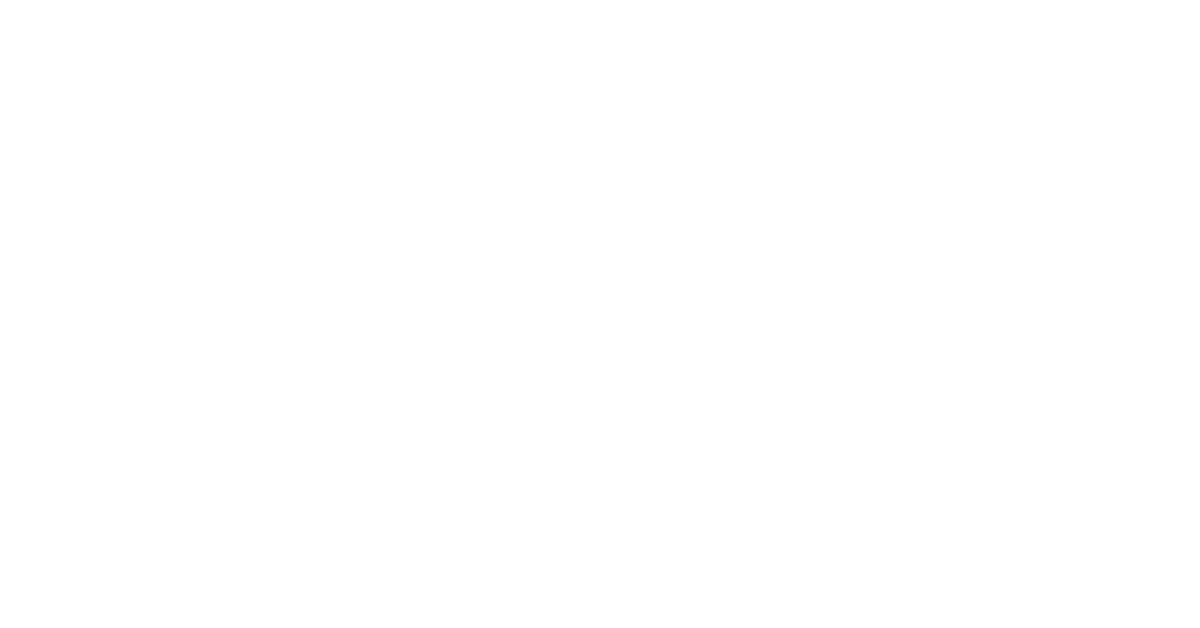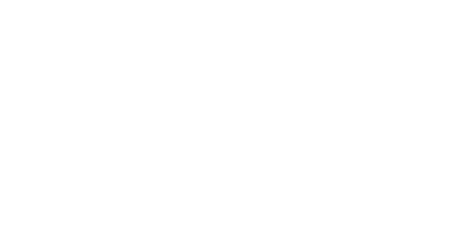# How To Reverse A String In C++?How To Reverse A String In C++?

## Introduction

Most of the competitive coding questions are loaded with different problems of reverse Strings, Arrays, Data Structures, and many more.

Reversing a string is one of the important problems that are famous across many competitive coding problem banks. The main theme of the article is to get a complete idea of how it works along with code for readers.

Let’s start with the basic definition of String. The string is an array of characters or a group of characters followed by a null character. The basic working of reserve a string is, for example, if the input is given as “Code”, the output of the code should be “edoC”.

There are many methods to reverse a string some of them are:

• Using Recursion
• Using Stack
• Using Swapping in the same string
• Using Inbuilt functions
• Using Another String/Temporary character
• Using the Constructor

Let’s discuss some of the methods in C++.## Using recursion

We used recursive functions to reverse a string from the below programs by using different conditions through if statements.

C++ Code:

``````#include <bits/stdc++.h>
using namespace std;

/* Function to print reverse of the passed string */
void reverse(string str)
{
if(str.size() == 0)
{
return;
}
reverse(str.substr(1));
cout << str;
}

int main()
{
string a = "CodingNinjas";
reverse(a);
return 0;
}``````

Output:
sajniNgnidoC

## Using Stack

In the below code, we are using a stack to reverse the string in C++.

Code:

``````// C++ program to reverse a string using stack
#include <bits/stdc++.h>
using namespace std;

// A structure to represent a stack
class Stack
{
public:
int top;
unsigned capacity;
char* array;
};

// function to create a stack of given
// capacity. It initializes size of stack as 0
Stack* createStack(unsigned capacity)
{
Stack* stack = new Stack();
stack->capacity = capacity;
stack->top = -1;
stack->array = new char[(stack->capacity * sizeof(char))];
return stack;
}

// Stack is full when top is equal to the last index
int isFull(Stack* stack)
{ return stack->top == stack->capacity - 1; }

// Stack is empty when top is equal to -1
int isEmpty(Stack* stack)
{ return stack->top == -1; }

// Function to add an item to stack.
// It increases top by 1
void push(Stack* stack, char item)
{
if (isFull(stack))
return;
stack->array[++stack->top] = item;
}

// Function to remove an item from the stack.
// It decreases top by 1
char pop(Stack* stack)
{
if (isEmpty(stack))
return -1;
return stack->array[stack->top--];
}

// A stack based function to reverse a string
void reverse(char str[])
{
// Create a stack of capacity
//equal to length of string
int n = strlen(str);
Stack* stack = createStack(n);

// Push all characters of string to stack
int i;
for (i = 0; i < n; i++)
push(stack, str[i]);

// Pop all characters of string and
// put them back to str
for (i = 0; i < n; i++)
str[i] = pop(stack);
}

int main()
{
char str[] = "CodingNinajs";

reverse(str);
cout << "The reversed string is " << str;

return 0;
}
``````

Output:
The reversed string is sjaniNgnidoC

## Using Inbuilt functions

The reverse() function directly reverses the given string, and this function is present in string.h library. The code for this function is inbuilt in the library, we need to just give a string as the input to the function.

Code:

``````// using reverse()
#include <bits/stdc++.h>
using namespace std;
int main()
{
string str = "CodingNinjas";

// Reverse str[begin..end]
reverse(str.begin(), str.end());

cout << str;
return 0;
}``````

Output:
sajniNgnidoC

## Using another String/ Temporary Character

The idea is to transfer the string to another string in a reverse manner. All we need is the size of the string.

The approach is to initialize a character array of the same size and start copying the elements of the input string from the end.

Note:
We have also covered a topic on how to convert Array into Strings in any programming language.

Code:

``````#include<iostream>
#include<stdio.h>
using namespace std;
int main()
{
char str, strTemp;
int len, i=0;
cout<<"Enter the String: ";
gets(str);
while(str[i]!='\0')
i++;
len = i;
strTemp[len] = '\0';
len--;
i = 0;
while(str[i]!='\0')
{
strTemp[len] = str[i];
i++;
len--;
}
i=0;
while(strTemp[i]!='\0')
{
str[i] = strTemp[i];
i++;
}
cout<<"\nReverse = "<<str;
cout<<endl;
return 0;
}``````

Input:
CodingNinjas

Output:
Reverse = sajniNgnidoC

## Constructor

In the below code, passing reverse iterators to the constructor returns us a reversed string.

Code:

``````// A simple C++ program to reverse string using constructor
#include <bits/stdc++.h>
using namespace std;
int main(){

string str = "CodingNinjas";

//Use of reverse iterators
string rev = string(str.rbegin(),str.rend());

cout<<rev<<endl;
return 0;
}``````

Output:
sajniNgnidoC

How do you reverse a string?

Reversing a string can be done in multiple ways. Some of them are using the reverse() function, using constructor, recursion, and stack.

Which inbuilt function is used to reverse a string in C++?

The “reverse()” function is used to reverse a string in C++. It is present in string.h library.

How do you reverse a string without a reverse function?

To reverse a string, there are many methods like recursion, stacks, constructors in different languages.

Why the string is immutable?

The String itself is immutable in Java.
The reason for this is that the = operator for strings is overloaded, takes the string literal as an argument, and then loops through the string literal copying each character into a mutable char array.

## Key Takeaways

This article briefs about how to reverse a string in C++. The blog described some methods like using the reverse() function and stack.

And then some of the programs using recursion, constructors in C++, and finally Stack using different strings and characters to reverse the string.

Practicing more problems on Arrays and Strings helps to crack the interviews in top product-based companies

On an ending note, don’t forget that regular practice leads to mastery. So, don’t forget to use CodeStudio to practice the vast range of DSA problems frequently asked in interview rounds.

This will assist you in mastering efficient coding methodologies, with the added benefit of interview experiences from scholars in large product-based organizations. On the endnote, you can also check out reversing a string using C for more methods.

By Dharni Mandla